# Class 12 Maths NCERT Solutions for Chapter 7 Integrals Exercise 7.10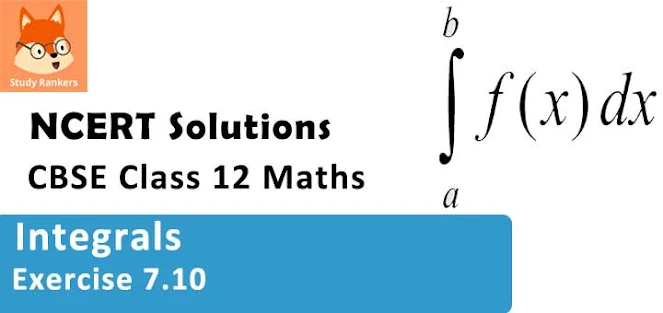### Integrals Exercise 7.10 Solutions

1. Evaluate the integrals in using substitution
01 x/(x2 + 1) dx

Solution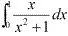Let x2 + 1 = t ⇒ 2x dx = dt
when x = 0, t = 1 and when x = 1, t = 2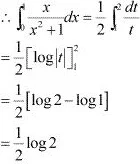2. Evaluate the integrals in using substitution
0π/2 √(sin Ø) cos5ф dф
Solution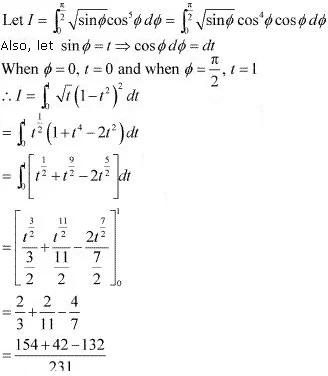= 64/231

3. ∫01 sin-1 [2x/(1 + x2)] dx
Solution
Consider, I = ∫01 sin-1 [2x/(1 + x2 )] dx
Let x = tan θ ⇒ dx = sec2 θ dθ
When x = 0, θ = 0 and when x = 1, θ = π/4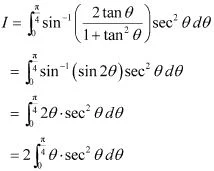Taking θ as first function and sec2 θ as second function and integrating by parts, we obtain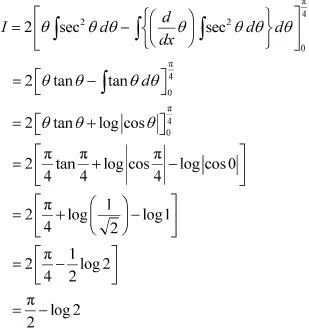4. Evaluate the integrals in using substitution ∫02 [x√(x + 2)] (Put x + 2 = t2
Solution
02 [x√(x + 2)]
Let x + 2 = t2 ⇒ dx = 2tdt
When x = 0, t = √2 and when x = 2, t = 2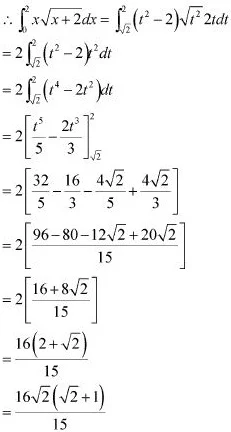5. Evaluate the integrals in using substitution ∫0π/2 sin x/(1 + cos2 x) dx
Solution
0π/2 sin x/(1 + cos2 x) dx
Let cos x = t ⇒ -sin x dx = dt
when x = 0, t = 1 and when x = π/2, t = 0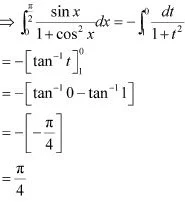6. Evaluate the integrals in using substitution  ∫02 dx/(x + 4 - x2)
Solution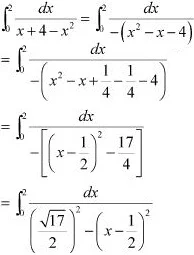Let x - 1/2 = t ⇒ dx = dt
When x = 0, t = -1/2 and when x = 2, t = 3/2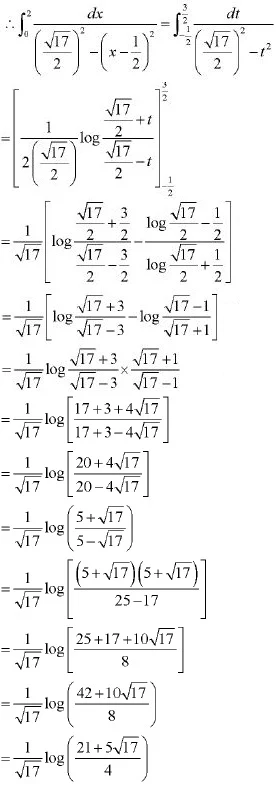7. Evaluate the integrals in using substitution ∫-11 dx/(x2 + 2x + 5)
Solution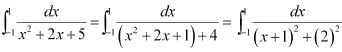Let x + 1 = t ⇒ dx = dt
When x = -1, t = 0 and when x = 1, t = 2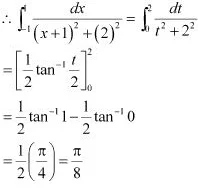8. Evaluate the integrals in using substitution ∫12 (1/x - 1/2x2) e2x dx
Solution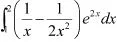Let 2x = t ⇒ 2dx = dt
When x = 1, t = 2 and when x = 2, t = 4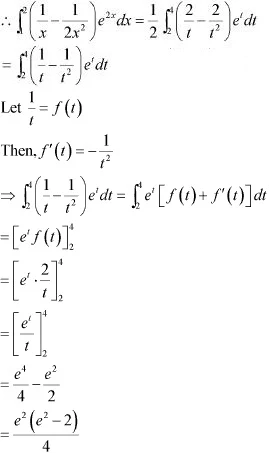9. The value of the integral  ∫1/34 [(x - x3 )1/3]/x4 dx is
(A) 6
(B) 0
(C) 3
(D) 4
Solution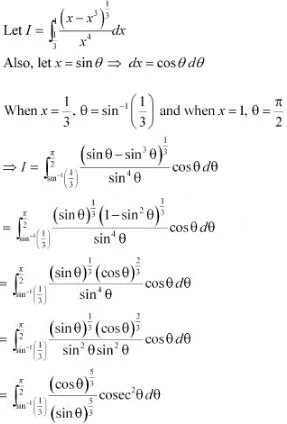Let cot θ = t ⇒ - cosec2 θ dθ = dt
When θ = sin-1 (1/3) . t = 2√2 and when θ = π/2 . t = 0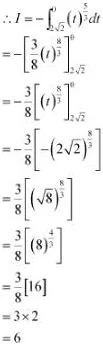Hence, the correct answer is A.

10. If f(x) = ∫0x t sin t dt, then f'(x) is
(A) cos x + x sin x
(B) x sin x
(C) x cos x
(D) sin x + x cos x
Solution
f(x) = ∫0x t sin t dt
Integrating by parts, we obtain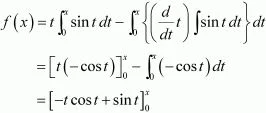= - x cos x + sin x
⇒ f'(x) = -[{x(-sin x)} + cos x] + cos x
= x sin x - cos x + cos x
= x sin x
Hence, the correct answer is B.